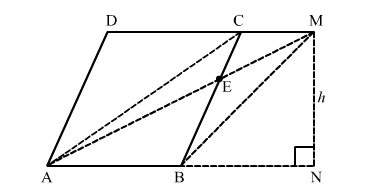# In a parallelogram ABCD, any point E is taken on the side BC.

Question:

In a parallelogram ABCD, any point E is taken on the side BCAE and DC when produced meet at a point M. Prove that

Solution:Join BM and AC.

$\operatorname{ar}(\Delta \mathrm{ADC})=\frac{1}{2} b h=\frac{1}{2} \times \mathrm{DC} \times h$

$\operatorname{ar}(\Delta \mathrm{ABM})=\frac{1}{2} \times \mathrm{AB} \times h$

AB = DC                               (Since ABCD is a parallelogram)